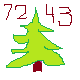Discussion about math, puzzles, games and fun.   Useful symbols: ÷ × ½ √ ∞ ≠ ≤ ≥ ≈ ⇒ ± ∈ Δ θ ∴ ∑ ∫  π  -¹ ² ³ °

You are not logged in.

## #1 2019-12-23 19:59:34

MKNB
Guest

### [Fast] Formula

Question How many bit strings of length 10 have
more 0s than 1s?

## #2 2020-01-20 12:17:55

John E. Franklin(2)
MemberRegistered: 2019-05-17
Posts: 20

### Re: [Fast] Formula

You can break the problem into five parts, for which can be totaled up for the answer.
1.) How many 10-bit binary strings have 4 ones?
2.) How many 10-bit binary strings have 3 ones?
3.) How many 10-bit binary strings have 2 ones?
4.) How many 10-bit binary strings have 1 one? Answer = ten
5.) How many 10-bit binary strings only zeros? Answer = one
So this is a start...  Sorry I don't know the rest off the bat.

Offline